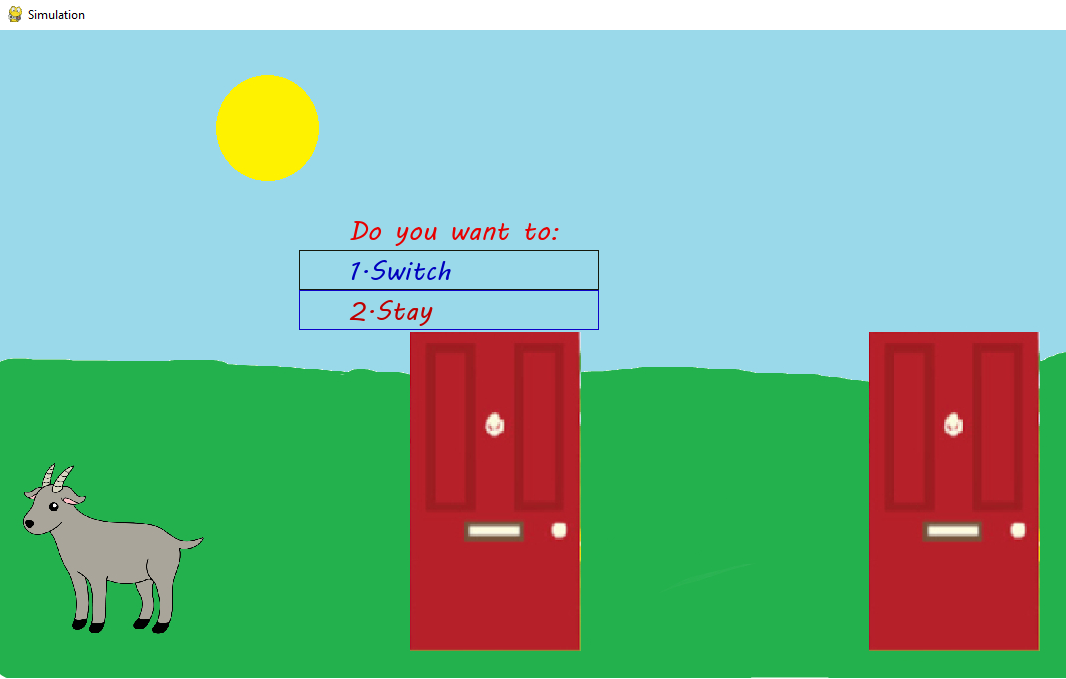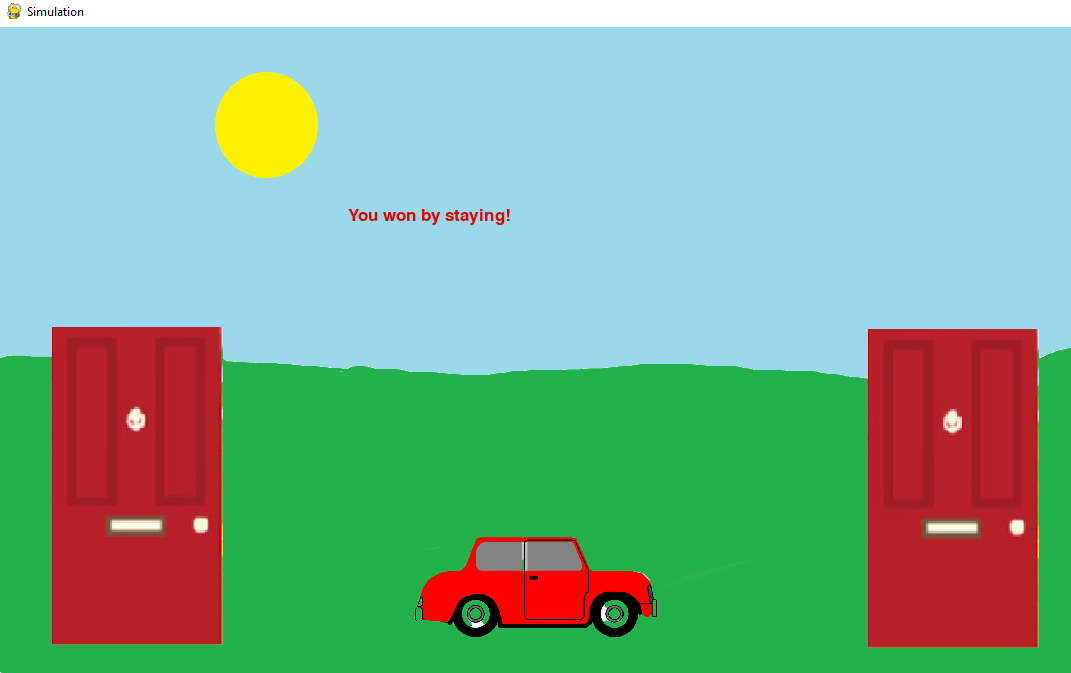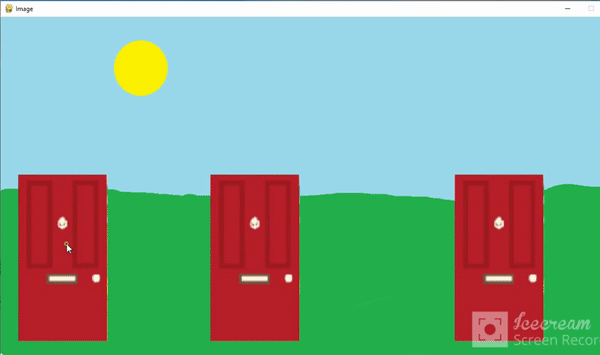# Monty Hall Problem’s Simulation Using Pygame

• Difficulty Level : Medium
• Last Updated : 17 Oct, 2021

In this article, we are going to see how to create Monty Hall games using Pygame in Python. Monty Hall was a game show of the American television game show Let’s Make a Deal.

Suppose you’re on a game show, and you’re given the choice of three doors, Behind one door is a car; behind the others, goats. You pick a door, say No. 1, and the host, who knows what’s behind the doors, opens another door, say No. 3, which has a goat. He then says to you, “Do you want to pick door No. 2?” Is it to your advantage to switch your choice?

Attention reader! Don’t stop learning now. Get hold of all the important DSA concepts with the DSA Self Paced Course at a student-friendly price and become industry ready.  To complete your preparation from learning a language to DS Algo and many more,  please refer Complete Interview Preparation Course.

In case you wish to attend live classes with experts, please refer DSA Live Classes for Working Professionals and Competitive Programming Live for Students.

Demo of what the end product would look like:The goat is revealed to behind door 1. Original selection was door 2.As the decision was made to stay, the game is won.

Please ensure that the images and the audio used are present in the same folder as the Python file. The images and the audio can be downloaded from here

## Work Flow and Logic

The entire simulation can be condensed into the following points:

• Since there are three doors, a random permutation of the numbers 1, 2, 3 would be generated, each number representing the door number. The first two numbers of this randomly generated permutation would correspond to the doors behind which goats are present, and the third number would be the door number behind which there is a car.
• Once the configuration is generated, the same is represented graphically through images. There is an image for each configuration.
• There is one point to be taken care of — according to the puzzle, after the user has chosen a door number, only the door behind which there is a goat is to be revealed. So, if the user selects the door behind which there is a car, then any of the two remaining doors can be revealed. However, if the user selects a door behind which there is a goat, only one of the two remaining doors can be revealed (as it is not permissible to reveal the door that the user has selected).

## Python3

 `import` `pygame``import` `random``pygame.init()``white ``=` `(``255``, ``255``, ``255``)``X ``=` `1200``Y ``=` `650``doors ``=` `random.sample(``range``(``1``, ``4``), ``3``)``goat1 ``=` `doors[``0``]``goat2 ``=` `doors[``1``]``goats ``=` `[goat1, goat2]``car ``=` `doors[``2``]`

Only two external modules are needed for this simulation pygame and random. At the beginning of every Pygame program, it is necessary to initialize Pygame by writing pygame.init(). The X and Y values refer to the dimensions of the window in which the simulation would run. These values can be altered based on the desired resolution and the images used. The list, doors, have values between 1 and 3 (inclusive). The order of these values would jumbled each time the program is run. This is done in order to assign the doors behind which there will be goats. According to the problem, there are a total of three doors behind two of these doors, there would be goats, and behind the third door, there would be a car. The variables goat1 and goat2 hold the door numbers behind which there would be goats. These numbers are then added to a list called goats. The third number is assigned to the variable, car.

## Python3

 `display_surface ``=` `pygame.display.set_mode((X, Y))``pygame.display.set_caption(``'Simulation'``)``image ``=` `pygame.image.load(``'all_doors.jpg'``)``change ``=` `False`` ` ` ` `def` `music():``    ``file` `=` `'click.mp3'``    ``pygame.mixer.init()``    ``pygame.mixer.music.load(``file``)``    ``pygame.mixer.music.play()`

The display_surface variable is like a canvas, upon which we would be adding entities (images, text etc.) as per the requirements. The argument passed through the display.set_caption function would be the title of the simulation window. The image that is to be shown at first — all three doors closed is loaded. A Boolean variable, change, is used to record changes. The function music is optional to have. Its function is to produce click sound whenever the user presses on a door. Be sure to have the mp3 file of whatever music is wished to be played. The function performs three steps initialize, load and play.

## Python3

 `def` `show_car(car, state):``    ``my_font ``=` `pygame.font.SysFont(``"latoblack"``, ``26``)``    ``display_surface ``=` `pygame.display.set_mode((X, Y))``    ``car1 ``=` `pygame.image.load(``'car_1.jpg'``)``    ``car2 ``=` `pygame.image.load(``'car_2.jpg'``)``    ``car3 ``=` `pygame.image.load(``'car_3.jpg'``)`` ` `    ``if` `car ``=``=` `1``:``        ``display_surface.blit(car1, (``0``, ``0``))``        ``pygame.display.update()``    ``elif` `car ``=``=` `2``:``        ``display_surface.blit(car2, (``0``, ``0``))``        ``pygame.display.update()``    ``elif` `car ``=``=` `3``:``        ``display_surface.blit(car3, (``0``, ``0``))``        ``pygame.display.update()``    ``if` `state ``=``=` `1``:``        ``the_text ``=` `my_font.render(``"You won by switching!"``, ``True``, (``231``, ``0``, ``10``))``        ``display_surface.blit(the_text, (``350``, ``180``))``        ``pygame.display.update()``    ``elif` `state ``=``=` `2``:``        ``the_text ``=` `my_font.render(``            ``"You could've won by staying!"``, ``True``, (``231``, ``0``, ``0``))``        ``display_surface.blit(the_text, (``350``, ``180``))``        ``pygame.display.update()``    ``elif` `state ``=``=` `3``:``        ``the_text ``=` `my_font.render(``"You won by staying!"``, ``True``, (``231``, ``0``, ``0``))``        ``display_surface.blit(the_text, (``350``, ``180``))``        ``pygame.display.update()``    ``elif` `state ``=``=` `4``:``        ``the_text ``=` `my_font.render(``            ``"You could've won by switcing!"``, ``True``, (``231``, ``0``, ``0``))``        ``display_surface.blit(the_text, (``350``, ``180``))``        ``pygame.display.update()`` ` ` ` `def` `draw_rect():``    ``pygame.draw.rect(display_surface, (``20``, ``24``, ``11``), ``                     ``(``300``, ``220``, ``300``, ``40``), ``1``)``    ``pygame.display.update()``    ``pygame.draw.rect(display_surface, (``14``, ``2``, ``200``),``                     ``(``300``, ``260``, ``300``, ``40``), ``1``)``    ``pygame.display.update()`

The show_car function may seem a bit cumbersome, but its functionality is fairly trivial. It aims to display the image of the appropriate orientation, i.e., if the car is behind door 3, the image corresponding to that orientation (car at position 3) would be displayed. All the images are available (along with the names used in the code) at the end of this article. The state parameter tells the outcome of the simulation.

There are four possible outcomes:

It is necessary to use pygame.display.update() for any display changes to take place. The font and font size can be altered by changing the parameters in the my_font variable. The first argument of display_surface.blit is the image that is to be rendered, and the second argument is a tuple denoting the X and Y coordinates. my_font has an attribute render used for displayed text. The first argument is the text that is to be displayed, and the third argument is a tuple containing RGB values for specifying the color of the text.  display_surface.blit is used to render the text specified by render onto the canvas. The first argument is the specifications of the text, and the second argument has the X and Y coordinates. In order to find the coordinates, use:

## Python3

 `x, y ``=` `pygame.mouse.get_pos()`

This will create a crosshair and the coordinates of the points clicked would be known.

## Python3

 `while` `True``:``    ``music()``    ``if` `change ``=``=` `False``:``        ``display_surface.fill(white)``        ``display_surface.blit(image, (``0``, ``0``))``    ``for` `event ``in` `pygame.event.get():``        ``if` `event.``type` `=``=` `pygame.QUIT:``            ``pygame.quit()``        ``pygame.display.update()``        ``clicked ``=` `False`` ` `        ``if` `event.``type` `=``=` `pygame.MOUSEBUTTONDOWN:``           ` `            ``# Check if door 1 is pressed.``            ``if``(event.pos[``0``] >``=` `71` `and` `event.pos[``0``] <``=` `203` `               ``and` `event.pos[``1``] >``=` `387` `and` `event.pos[``1``] <``=` `632``):``                ``user ``=` `1``                ``clicked ``=` `True``                ``music()``            ``# Check if door 2 is pressed.``            ``elif``(event.pos[``0``] >``=` `353` `and` `event.pos[``0``] <``=` `485` `                 ``and` `event.pos[``1``] >``=` `386` `and` `event.pos[``1``] <``=` `635``):``                ``user ``=` `2``                ``clicked ``=` `True``                ``music()``            ``# Check if door 3 is pressed.``            ``elif``(event.pos[``0``] >``=` `938` `and` `event.pos[``0``] <``=` `1100` `                 ``and` `event.pos[``1``] >``=` `387` `and` `event.pos[``1``] <``=` `633``):``                ``user ``=` `3``                ``# print("Clicked on door 3.")``                ``clicked ``=` `True``                ``music()`

Every game contains an infinite loop (while True). It is checked if the type of event registered is QUIT, i.e. the user has closed the Pygame window. In that case, the program terminates. pygame.MOUSEBUTTONDOWN refers to the event of left-clicking a mouse. The if conditions are used to determine the door that has been pressed by the user (based on the coordinates) and the door number is assigned to the variable, user. For example, if the X coordinate (event.pos) of the point where the click has occurred is between 71 and 203, and the Y coordinate (event.pos) is between 387 and 633, register the click has a click on door 1. The clicked Boolean is set to True whenever one of the three doors is clicked.

## Python3

 `if` `clicked:``            ``image1 ``=` `pygame.image.load(``'goat_1.jpg'``)``            ``image2 ``=` `pygame.image.load(``'goat_2.jpg'``)``            ``image3 ``=` `pygame.image.load(``'goat_3.jpg'``)``            ``image4 ``=` `pygame.image.load(``'car_1.jpg'``)``            ``image5 ``=` `pygame.image.load(``'car_2.jpg'``)``            ``image6 ``=` `pygame.image.load(``'car_3.jpg'``)``            ``wr ``=` `random.randint(``0``, ``1``)``            ``if``(goats[``0``] ``=``=` `user):``                ``g ``=` `goats[``1``]``            ``elif``(goats[``1``] ``=``=` `user):``                ``g ``=` `goats[``0``]``            ``else``:``                ``g ``=` `goats[wr]``            ``if` `g ``=``=` `1``:``                ``change ``=` `True``                ``display_surface.blit(image1, (``0``, ``0``))``                ``pygame.display.update()``            ``elif` `g ``=``=` `2``:``                ``change ``=` `True``                ``display_surface.blit(image2, (``0``, ``0``))``                ``pygame.display.update()``            ``elif` `g ``=``=` `3``:``                ``change ``=` `True``                ``display_surface.blit(image3, (``0``, ``0``))``                ``pygame.display.update()``            ``print``(u``"There is a goat behind door {}"``.``format``(g))``            ``my_font ``=` `pygame.font.SysFont(``"mvboli"``, ``26``)``            ``the_text ``=` `my_font.render(``"Do you want to:"``, ``True``, (``231``, ``0``, ``0``))``            ``display_surface.blit(the_text, (``350``, ``180``))``            ``the_text2 ``=` `my_font.render(``"1.Switch"``, ``True``, (``0``, ``0``, ``190``))``            ``display_surface.blit(the_text2, (``350``, ``220``))``            ``the_text3 ``=` `my_font.render(``"2.Stay"``, ``True``, (``190``, ``0``, ``0``))``            ``display_surface.blit(the_text3, (``350``, ``260``))``            ``draw_rect()``            ``clicked2 ``=` `False``            ``print``(u``"The car is behind door {}"``.``format``(car))`` ` ` ` `        ``clicked2 ``=` `False``        ``if` `event.``type` `=``=` `pygame.MOUSEBUTTONDOWN:``          ``# Compare click coordinates with``          ``# coordinates where it says 'Switch' and 'Stay'.``            ``if``(event.pos[``0``] >``=` `299` `and` `event.pos[``0``] <``=` `597` `               ``and` `event.pos[``1``] >``=` `220` `               ``and` `event.pos[``1``] <``=` `260``):``               ` `                  ``# user2 = 1 means user has chosen to switch.``                ``user2 ``=` `1`  `                ``clicked2 ``=` `True``            ``elif``(event.pos[``0``] >``=` `301` `and` `event.pos[``0``] <``=` `598` `                 ``and` `event.pos[``1``] >``=` `259` `and` `event.pos[``1``] <``=` `297``):``                ``user2 ``=` `2`   `# user2 = 2 means user has chosen to stay. ``                ``clicked2 ``=` `True`

Once a valid click is registered, the six images are loaded. it is checked if the user has clicked on a door that has a goat behind it. As the door selected by the user cannot be opened and the door that can be opened must contain a goat behind it, there is only one possible door that can be opened. Say, goats are behind doors 1 and 2, and the user clicks on door 1, as door 1 cannot be opened, door 2 is the only door that can be opened. The variable, g, stores the valid door that can be opened. If the user did not select a door that has a goat behind it, a goat is randomly assigned using the randint function, and storing either 1 or 0 in the variable, wr

The variable, user2, stores whether the user chose to stay or switch. The Boolean, clicked2, stores whether the click for determining the choice (stay or switch) has been registered or not.

## Python3

 `if` `clicked2:`` ` `    ``if` `user2 ``=``=` `1``:``        ``print``(``"You chose to switch!"``)``        ``if` `user ``in` `goats:``            ``my_font ``=` `pygame.font.SysFont(``"mvboli"``, ``26``)``            ``the_text ``=` `my_font.render(``                ``"You won by switching!"``, ``True``, (``231``, ``0``, ``0``))``            ``state ``=` `1``            ``display_surface.blit(the_text, (``350``, ``180``))``            ``pygame.display.update()``            ``print``(``"You won by switching!"``)``        ``else``:   ``# User has chosen the door behind which there is a car.``            ``my_font ``=` `pygame.font.SysFont(``"mvboli"``, ``26``)``            ``the_text2 ``=` `my_font.render(``                ``"You could've won by staying!"``, ``True``, (``231``, ``0``, ``0``))``            ``state ``=` `2``            ``display_surface.blit(the_text2, (``350``, ``180``))``            ``pygame.display.update()``            ``print``(``"You could have won by switching!"``)``    ``elif` `user2 ``=``=` `2``:``        ``print``(``"You chose to stay!"``)``        ``if` `user ``=``=` `car:``            ``my_font ``=` `pygame.font.SysFont(``"mvboli"``, ``26``)``            ``the_text3 ``=` `my_font.render(``                ``"You won by staying!"``, ``True``, (``231``, ``0``, ``0``))``            ``display_surface.blit(the_text3, (``350``, ``180``))``            ``state ``=` `3``            ``pygame.display.update()``            ``print``(``"You won by staying!"``)``        ``else``:``            ``my_font ``=` `pygame.font.SysFont(``"mvboli"``, ``26``)``            ``the_text4 ``=` `my_font.render(``                ``"You could've won by switching!"``, ``True``, (``231``, ``0``, ``0``))``            ``display_surface.blit(the_text4, (``350``, ``180``))``            ``state ``=` `4``            ``pygame.display.update()``            ``print``(``"You could have won by switching!"``)``    ``show_car(car, state)`

Once the user has selected, it is determined whether they have won or not (whether there is a car behind the door they ultimately chose). Determining the winner can be understood by the following table:

## Python3

 `import` `pygame``import` `random``pygame.init()``white ``=` `(``255``, ``255``, ``255``)``X ``=` `1200``Y ``=` `650``doors ``=` `random.sample(``range``(``1``, ``4``), ``3``)``goat1 ``=` `doors[``0``]``goat2 ``=` `doors[``1``]``goats ``=` `[goat1, goat2]``car ``=` `doors[``2``]``display_surface ``=` `pygame.display.set_mode((X, Y))``pygame.display.set_caption(``'Simulation'``)``image ``=` `pygame.image.load(``'all_doors.jpg'``)``change ``=` `False``msg_disp ``=` `False`` ` ` ` `def` `music():``    ``file` `=` `'click.mp3'``    ``pygame.mixer.init()``    ``pygame.mixer.music.load(``file``)``    ``pygame.mixer.music.play()`` ` ` ` `def` `show_car(car, state):``    ``my_font ``=` `pygame.font.SysFont(``"latoblack"``, ``26``)``    ``display_surface ``=` `pygame.display.set_mode((X, Y))``    ``car1 ``=` `pygame.image.load(``'car_1.jpg'``)``    ``car2 ``=` `pygame.image.load(``'car_2.jpg'``)``    ``car3 ``=` `pygame.image.load(``'car_3.jpg'``)`` ` `    ``if` `car ``=``=` `1``:``        ``display_surface.blit(car1, (``0``, ``0``))``        ``pygame.display.update()``    ``elif` `car ``=``=` `2``:``        ``display_surface.blit(car2, (``0``, ``0``))``        ``pygame.display.update()``    ``elif` `car ``=``=` `3``:``        ``display_surface.blit(car3, (``0``, ``0``))``        ``pygame.display.update()``    ``if` `state ``=``=` `1``:``        ``the_text ``=` `my_font.render(``"You won by switching!"``,``                                  ``True``, (``231``, ``0``, ``10``))``        ``display_surface.blit(the_text, (``350``, ``180``))``        ``pygame.display.update()``    ``elif` `state ``=``=` `2``:``        ``the_text ``=` `my_font.render(``            ``"You could've won by staying!"``, ``True``,``          ``(``231``, ``0``, ``0``))``        ``display_surface.blit(the_text, (``350``, ``180``))``        ``pygame.display.update()``    ``elif` `state ``=``=` `3``:``        ``the_text ``=` `my_font.render(``"You won by staying!"``, ``                                  ``True``, (``231``, ``0``, ``0``))``        ``display_surface.blit(the_text, (``350``, ``180``))``        ``pygame.display.update()``    ``elif` `state ``=``=` `4``:``        ``the_text ``=` `my_font.render(``            ``"You could've won by switcing!"``, ``True``,``          ``(``231``, ``0``, ``0``))``        ``display_surface.blit(the_text, (``350``, ``180``))``        ``pygame.display.update()`` ` ` ` `def` `draw_rect():``    ``pygame.draw.rect(display_surface, (``20``, ``24``, ``11``), ``                     ``(``300``, ``220``, ``300``, ``40``), ``1``)``    ``pygame.display.update()``    ``pygame.draw.rect(display_surface, (``14``, ``2``, ``200``), ``                     ``(``300``, ``260``, ``300``, ``40``), ``1``)``    ``pygame.display.update()`` ` ` ` `while` `True``:``    ``music()``    ``if` `change ``=``=` `False``:``        ``display_surface.fill(white)``        ``display_surface.blit(image, (``0``, ``0``))``    ``for` `event ``in` `pygame.event.get():``        ``if` `event.``type` `=``=` `pygame.QUIT:``            ``pygame.quit()`` ` `        ``pygame.display.update()`` ` `        ``clicked ``=` `False`` ` `        ``if` `event.``type` `=``=` `pygame.MOUSEBUTTONDOWN:``            ``# Check if door 1 is pressed.``            ``if``(event.pos[``0``] >``=` `71` `and` `event.pos[``0``] <``=` `203``               ``and` `event.pos[``1``] >``=` `387` `and` `event.pos[``1``] <``=` `632``):``                ``user ``=` `1``                ``clicked ``=` `True``                ``music()``            ``# Check if door 2 is pressed.``            ``elif``(event.pos[``0``] >``=` `353` `and` `event.pos[``0``] <``=` `485` `                 ``and` `event.pos[``1``] >``=` `386` `and` `event.pos[``1``] <``=` `635``):``                ``user ``=` `2``                ``clicked ``=` `True``                ``music()``            ``# Check if door 3 is pressed.``            ``elif``(event.pos[``0``] >``=` `938` `and` `event.pos[``0``] <``=` `1100` `                 ``and` `event.pos[``1``] >``=` `387` `and` `event.pos[``1``] <``=` `633``):``                ``user ``=` `3``                 ` `                ``clicked ``=` `True``                ``music()``        ``if` `clicked:`` ` `            ``image1 ``=` `pygame.image.load(``'goat_1.jpg'``)``            ``image2 ``=` `pygame.image.load(``'goat_2.jpg'``)``            ``image3 ``=` `pygame.image.load(``'goat_3.jpg'``)``            ``image4 ``=` `pygame.image.load(``'car_1.jpg'``)``            ``image5 ``=` `pygame.image.load(``'car_2.jpg'``)``            ``image6 ``=` `pygame.image.load(``'car_3.jpg'``)``            ``wr ``=` `random.randint(``0``, ``1``)``            ``if``(goats[``0``] ``=``=` `user):``                ``g ``=` `goats[``1``]``            ``elif``(goats[``1``] ``=``=` `user):``                ``g ``=` `goats[``0``]``            ``else``:``                ``g ``=` `goats[wr]``            ``if` `g ``=``=` `1``:``                ``change ``=` `True``                ``display_surface.blit(image1, (``0``, ``0``))``                ``pygame.display.update()``            ``elif` `g ``=``=` `2``:``                ``change ``=` `True``                ``display_surface.blit(image2, (``0``, ``0``))``                ``pygame.display.update()``            ``elif` `g ``=``=` `3``:``                ``change ``=` `True``                ``display_surface.blit(image3, (``0``, ``0``))``                ``pygame.display.update()``            ``print``(u``"There is a goat behind door {}"``.``format``(g))`` ` `            ``my_font ``=` `pygame.font.SysFont(``"mvboli"``, ``26``)``            ``the_text ``=` `my_font.render(``"Do you want to:"``, ``True``, (``231``, ``0``, ``0``))``            ``display_surface.blit(the_text, (``350``, ``180``))``            ``the_text2 ``=` `my_font.render(``"1.Switch"``, ``True``, (``0``, ``0``, ``190``))``            ``display_surface.blit(the_text2, (``350``, ``220``))``            ``the_text3 ``=` `my_font.render(``"2.Stay"``, ``True``, (``190``, ``0``, ``0``))``            ``display_surface.blit(the_text3, (``350``, ``260``))``            ``draw_rect()``            ``clicked2 ``=` `False``            ``print``(u``"The car is behind door {}"``.``format``(car))`` ` `    ``# for event in pygame.event.get():``        ``clicked2 ``=` `False``        ``if` `event.``type` `=``=` `pygame.MOUSEBUTTONDOWN:``           ` `            ``# Compare click coordinates with coordinates``            ``# where it says 'Switch' and 'Stay'.``            ``if``(event.pos[``0``] >``=` `299` `and` `event.pos[``0``] <``=` `597``               ``and` `event.pos[``1``] >``=` `220` `and` `event.pos[``1``] <``=` `260``):``                   ` `                ``# user2 = 1 means user has chosen to switch.``                ``user2 ``=` `1`  `                ``clicked2 ``=` `True``            ``elif``(event.pos[``0``] >``=` `301` `and` `event.pos[``0``] <``=` `598` `                 ``and` `event.pos[``1``] >``=` `259` `and` `event.pos[``1``] <``=` `297``):``               ` `                  ``# user2 = 2 means user has chosen to stay.``                ``user2 ``=` `2`   `                ``clicked2 ``=` `True`` ` `        ``if` `clicked2:`` ` `            ``if` `user2 ``=``=` `1``:``                ``print``(``"You chose to switch!"``)``                ``if` `user ``in` `goats:``                    ``my_font ``=` `pygame.font.SysFont(``"mvboli"``, ``26``)``                    ``the_text ``=` `my_font.render(``                        ``"You won by switching!"``, ``True``, (``231``, ``0``, ``0``))``                    ``state ``=` `1``                    ``display_surface.blit(the_text, (``350``, ``180``))``                    ``pygame.display.update()``                    ``print``(``"You won by switching!"``)``                     ` `                ``# User has chosen the door behind which there is a car.``                ``else``:   ``                    ``my_font ``=` `pygame.font.SysFont(``"mvboli"``, ``26``)``                    ``the_text2 ``=` `my_font.render(``                        ``"You could've won by staying!"``, ``True``, (``231``, ``0``, ``0``))``                    ``state ``=` `2``                    ``display_surface.blit(the_text2, (``350``, ``180``))``                    ``pygame.display.update()``                    ``print``(``"You could have won by switching!"``)``            ``elif` `user2 ``=``=` `2``:``                ``print``(``"You chose to stay!"``)``                ``if` `user ``=``=` `car:``                    ``my_font ``=` `pygame.font.SysFont(``"mvboli"``, ``26``)``                    ``the_text3 ``=` `my_font.render(``                        ``"You won by staying!"``, ``True``, (``231``, ``0``, ``0``))``                    ``display_surface.blit(the_text3, (``350``, ``180``))``                    ``state ``=` `3``                    ``pygame.display.update()``                    ``print``(``"You won by staying!"``)``                ``else``:``                    ``my_font ``=` `pygame.font.SysFont(``"mvboli"``, ``26``)``                    ``the_text4 ``=` `my_font.render(``                        ``"You could've won by switching!"``, ``True``, (``231``, ``0``, ``0``))``                    ``display_surface.blit(the_text4, (``350``, ``180``))``                    ``state ``=` `4``                    ``pygame.display.update()``                    ``print``(``"You could have won by switching!"``)``            ``show_car(car, state)`

Output:My Personal Notes arrow_drop_up